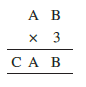# Q5.Find the values of the letters in each of the following and give reasons for the steps involved. .Here multiplication of 3 and B gives  a number whose unit place digit is B .

Possible value of B = 0 and 5

let B = 5

3 * A  + 1 = CA

this is not possible for any value of A.

Hence B = 0

now  A * 3 = CA ( a number whose unit place digit is A itself when multiplied by 3) hence

possible value of A = 5 and 0

since AB is a two digit number A can not equal to 0.

hence A = 5

A * 3 + 1 = CA

5 * 3 + 1 = 15

hence C = 1

A = 5, B = 5 and C = 1.

## Related Chapters

### Preparation Products

##### Knockout JEE Main April 2021

An exhaustive E-learning program for the complete preparation of JEE Main..

₹ 22999/- ₹ 14999/-
##### Knockout NEET May 2021

An exhaustive E-learning program for the complete preparation of NEET..

₹ 22999/- ₹ 14999/-
##### Knockout JEE Main April 2022

An exhaustive E-learning program for the complete preparation of JEE Main..

₹ 34999/- ₹ 24999/-
##### Test Series NEET May 2021

Take chapter-wise, subject-wise and Complete syllabus mock tests and get in depth analysis of your test..

₹ 6999/- ₹ 4999/-